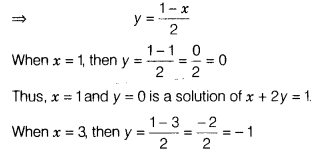# Write two solutions of the linear equation x + 2y = 1

Write two solutions of the linear equation
x + 2y = 1.

The given equation x + 2y = 1 can be written as
2y = 1 - xThus.x = 3 and y = -1 is another solution of x + 2y = 1.
Hence, the two solutions of x + 2y = 1, out of infinitely solutions, are (1,0) and (3, -1).